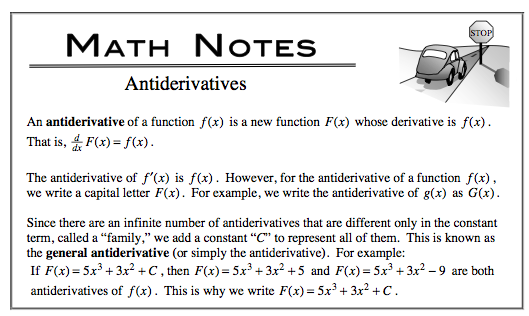### Home > CALC > Chapter 7 > Lesson 7.2.4 > Problem7-99

7-99.

Find a possible equation for $y$ given $\frac { d y } { d x }= 12e^{4x} −\frac { 1 } { x }$.

You know the derivative. But you want to know the original function. What should you do?